# Basic embedding with EmbedSOM

## Dataset

We will embed a small dataset created from gaussian clusters positioned in vertices of a 5-dimensional hypercube.

#create the seed dataset
n <- 1024
data <- matrix(c(rep(0,n),rep(1,n)),ncol=1)

for(i in 2:5) data <- cbind(c(rep(0,dim(data)), rep(1, dim(data))),rbind(data,data))

#scatter the points to clusters
set.seed(1)
data <- data + 0.2*rnorm(dim(data)*dim(data))
colnames(data) <- paste0('V',1:5)


This looks relatively nicely from the side (each corner in fact hides 8 separate clusters):

plot(data, pch=19, col=rgb(0,0,0,0.2))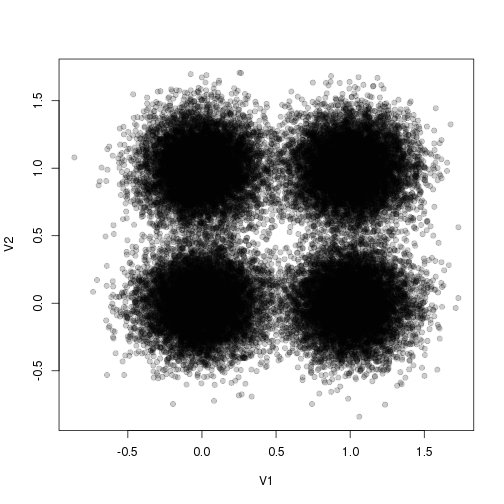Linear dimensionality reduction doesn't help much with seeing all 32 clusters:

plot(data.frame(prcomp(data)\$x), pch='.', col=rgb(0,0,0,0.2))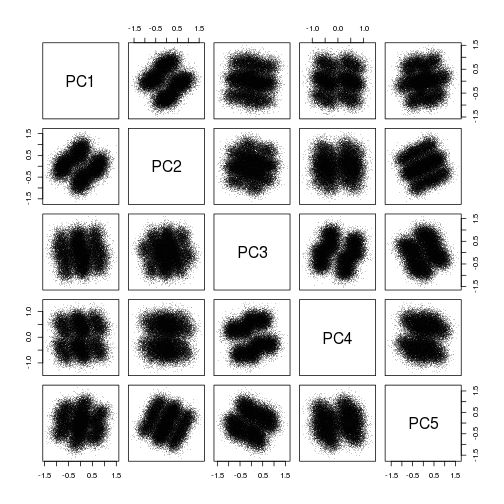Let's use the non-linear EmbedSOM instead.

EmbedSOM works on a self-organizing map that you need to create first:

set.seed(1)
map <- EmbedSOM::SOM(data, xdim=24, ydim=24)


EmbedSOM provides some level of compatibility with FlowSOM that can be used to simplify some commands. FlowSOM-originating maps and whole FlowSOM object may be used as well:

fs <- FlowSOM::ReadInput(as.matrix(data.frame(data)))
fs <- FlowSOM::BuildSOM(fsom=fs, xdim=24, ydim=24)


$$24\times24$$ is the recommended SOM size for getting something interesting from EmbedSOM – it provides a good amount of detail, and still runs quite quickly.

## Embedding

When the SOM is ready, a matrix of 2-dimensional coordinates is obtained using the EmbedSOM function:

e <- EmbedSOM::EmbedSOM(data=data, map=map)


Alternatively, FlowSOM objects are supported to be used instead of data and map parameters in most EmbedSOM commands:

e <- EmbedSOM::EmbedSOM(fsom=fs)


Several extra parameters may be specified; e.g. the following code makes the embedding a bit smoother and faster (but not necessarily better). See the EmbedSOM paper for details on parameters.

e <- EmbedSOM::EmbedSOM(data=data, map=map, smooth=2, k=10)


Finally, e now contains the dimensionality-reduced 2D coordinates of the original data that can be used for plotting.

head(e)

##      EmbedSOM1 EmbedSOM2
## [1,]  23.47801  13.42236
## [2,]  22.86703  12.98544
## [3,]  23.63919  14.31299
## [4,]  21.65178  12.51104
## [5,]  22.58825  13.94369
## [6,]  23.12144  13.64124


## Plotting the data

The embedding can be plotted using the standard graphics function, nicely showing all clusters next to each other.

plot(e, pch=19, cex=.5, col=rgb(0,0,0,0.2))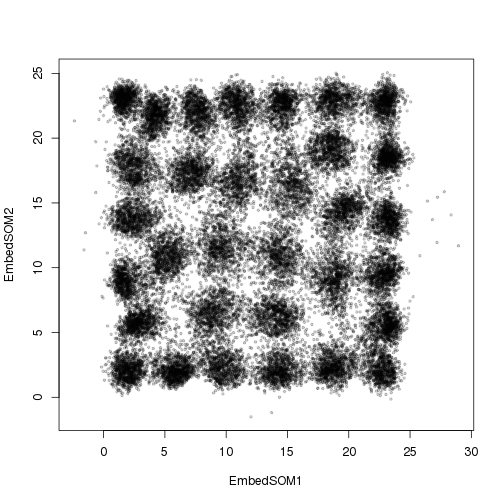EmbedSOM provides specialized plotting function which is useful in many common use cases; for example for displaying density:

EmbedSOM::PlotEmbed(e, pch=19, cex=.5, nbin=100)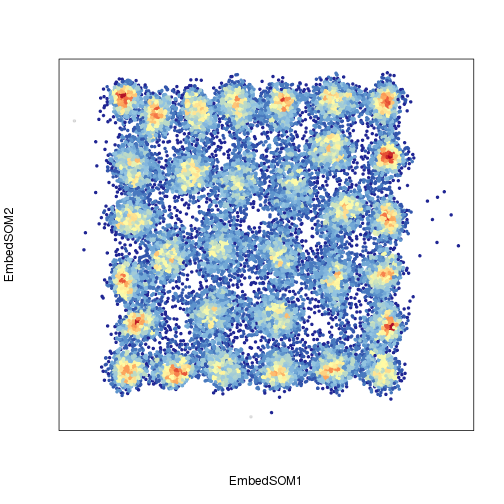Or for seeing colored expression of a single marker (value=1 specifies a column number; column names can be used as well):

EmbedSOM::PlotEmbed(e, data=data, pch=19, cex=.5, alpha=0.3, value=1)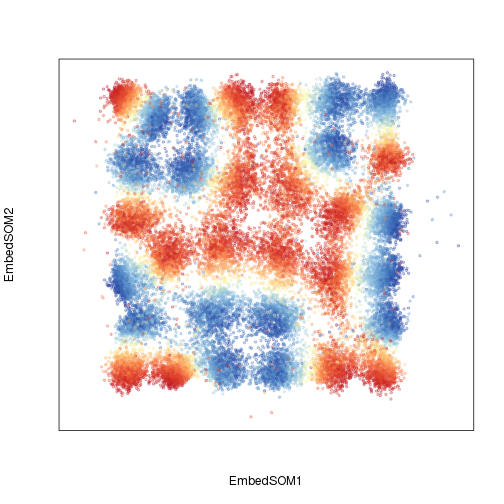(Notice that it is necessary to pass in the original data frame. When working with FlowSOM, the same can be done using fsom=fs.)

Or multiple markers:

EmbedSOM::PlotEmbed(e, data=data, pch=19, cex=.5, alpha=0.3, red=2, green=4)